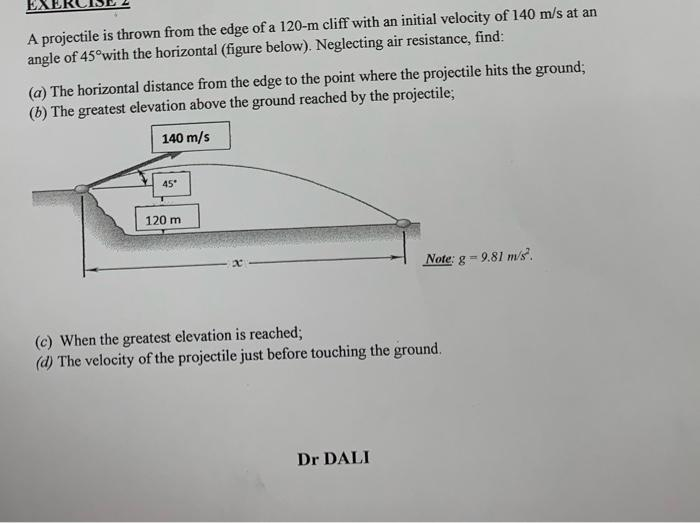Home / Expert Answers / Mechanical Engineering / a-projectile-is-thrown-from-the-edge-of-a-120m-cliff-with-an-initial-velocity-of-140m-s-at-an-pa154

# (Solved): A projectile is thrown from the edge of a 120m cliff with an initial velocity of 140m/s at an ...A projectile is thrown from the edge of a cliff with an initial velocity of at an angle of with the horizontal (figure below). Neglecting air resistance, find: (a) The horizontal distance from the edge to the point where the projectile hits the ground; (h) The greatest elevation above the ground reached by the projectile; (c) When the greatest elevation is reached; (d) The velocity of the projectile just before touching the ground.

We have an Answer from Expert